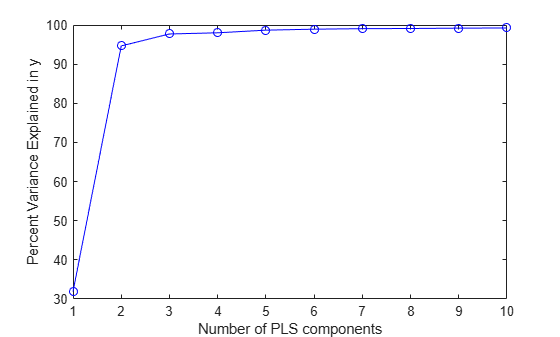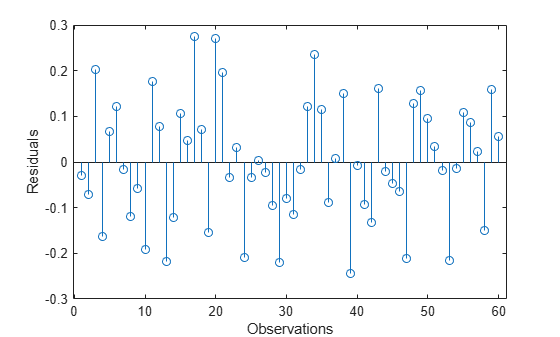# plsregress

Partial least-squares regression

## Syntax

```[XL,YL] = plsregress(X,Y,ncomp) [XL,YL,XS] = plsregress(X,Y,ncomp) [XL,YL,XS,YS] = plsregress(X,Y,ncomp) [XL,YL,XS,YS,BETA] = plsregress(X,Y,ncomp,...) [XL,YL,XS,YS,BETA,PCTVAR] = plsregress(X,Y,ncomp) [XL,YL,XS,YS,BETA,PCTVAR,MSE] = plsregress(X,Y,ncomp) [XL,YL,XS,YS,BETA,PCTVAR,MSE] = plsregress(...,param1,val1,param2,val2,...) [XL,YL,XS,YS,BETA,PCTVAR,MSE,stats] = plsregress(X,Y,ncomp,...) ```

## Description

`[XL,YL] = plsregress(X,Y,ncomp)` computes a partial least-squares (PLS) regression of `Y` on `X`, using `ncomp` PLS components, and returns the predictor and response loadings in `XL` and `YL`, respectively. `X` is an n-by-p matrix of predictor variables, with rows corresponding to observations and columns to variables. `Y` is an n-by-m response matrix. `XL` is a p-by-`ncomp` matrix of predictor loadings, where each row contains coefficients that define a linear combination of PLS components that approximate the original predictor variables. `YL` is an m-by-`ncomp` matrix of response loadings, where each row contains coefficients that define a linear combination of PLS components that approximate the original response variables.

`[XL,YL,XS] = plsregress(X,Y,ncomp)` returns the predictor scores `XS`, that is, the PLS components that are linear combinations of the variables in `X`. `XS` is an n-by-`ncomp` orthonormal matrix with rows corresponding to observations and columns to components.

`[XL,YL,XS,YS] = plsregress(X,Y,ncomp)` returns the response scores `YS`, that is, the linear combinations of the responses with which the PLS components `XS` have maximum covariance. `YS` is an n-by-`ncomp` matrix with rows corresponding to observations and columns to components. `YS` is neither orthogonal nor normalized.

`plsregress` uses the SIMPLS algorithm, first centering `X` and `Y` by subtracting off column means to get centered variables `X0` and `Y0`. However, it does not rescale the columns. To perform PLS with standardized variables, use `zscore` to normalize `X` and `Y`.

If `ncomp` is omitted, its default value is `min(size(X,1)-1,size(X,2))`.

The relationships between the scores, loadings, and centered variables `X0` and `Y0` are:

`XL = (XS\X0)' = X0'*XS`,

`YL = (XS\Y0)' = Y0'*XS`,

`XL` and `YL` are the coefficients from regressing `X0` and `Y0` on `XS`, and `XS*XL'` and `XS*YL'` are the PLS approximations to `X0` and `Y0`.

`plsregress` initially computes `YS` as:

`YS = Y0*YL = Y0*Y0'*XS`,

By convention, however, `plsregress` then orthogonalizes each column of `YS` with respect to preceding columns of `XS`, so that `XS'*YS` is lower triangular.

`[XL,YL,XS,YS,BETA] = plsregress(X,Y,ncomp,...)` returns the PLS regression coefficients `BETA`. `BETA` is a (p+1)-by-m matrix, containing intercept terms in the first row:

`Y = [ones(n,1),X]*BETA + Yresiduals`,

`Y0 = X0*BETA(2:end,:) + Yresiduals`. Here `Yresiduals` is the vector of response residuals.

`[XL,YL,XS,YS,BETA,PCTVAR] = plsregress(X,Y,ncomp)` returns a 2-by-`ncomp` matrix `PCTVAR` containing the percentage of variance explained by the model. The first row of `PCTVAR` contains the percentage of variance explained in `X` by each PLS component, and the second row contains the percentage of variance explained in `Y`.

`[XL,YL,XS,YS,BETA,PCTVAR,MSE] = plsregress(X,Y,ncomp)` returns a 2-by-(`ncomp`+1) matrix `MSE` containing estimated mean-squared errors for PLS models with `0:ncomp` components. The first row of `MSE` contains mean-squared errors for the predictor variables in `X`, and the second row contains mean-squared errors for the response variable(s) in `Y`.

`[XL,YL,XS,YS,BETA,PCTVAR,MSE] = plsregress(...,param1,val1,param2,val2,...)` specifies optional parameter name/value pairs from the following table to control the calculation of `MSE`.

ParameterValue
`'cv'`

The method used to compute `MSE`.

• When the value is a positive integer `k`, `plsregress` uses `k`-fold cross-validation.

• When the value is an object of the `cvpartition` class, other forms of cross-validation can be specified.

• When the value is `'resubstitution'`, `plsregress` uses `X` and `Y` both to fit the model and to estimate the mean-squared errors, without cross-validation.

The default is `'resubstitution'`.

`'mcreps'`

A positive integer indicating the number of Monte-Carlo repetitions for cross-validation. The default value is `1`. The value must be `1` if the value of `'cv'` is `'resubstitution'`.

`options`

A structure that specifies whether to run in parallel, and specifies the random stream or streams. Create the `options` structure with `statset`. Option fields:

• `UseParallel` — Set to `true` to compute in parallel. Default is `false`.

• `UseSubstreams` — Set to `true` to compute in parallel in a reproducible fashion. Default is `false`. To compute reproducibly, set `Streams` to a type allowing substreams: `'mlfg6331_64'` or `'mrg32k3a'`.

• `Streams` — A `RandStream` object or cell array consisting of one such object. If you do not specify `Streams`, `plsregress` uses the default stream.

To compute in parallel, you need Parallel Computing Toolbox™

`[XL,YL,XS,YS,BETA,PCTVAR,MSE,stats] = plsregress(X,Y,ncomp,...)` returns a structure `stats` with the following fields:

• `W` — A p-by-`ncomp` matrix of PLS weights so that `XS = X0*W`.

• `T2` — The T2 statistic for each point in `XS`.

• `Xresiduals` — The predictor residuals, that is, `X0-XS*XL'`.

• `Yresiduals` — The response residuals, that is, `Y0-XS*YL'`.

## Examples

collapse all

Load data on near infrared (NIR) spectral intensities of 60 samples of gasoline at 401 wavelengths, and their octane ratings.

```load spectra X = NIR; y = octane;```

Perform PLS regression with ten components.

`[XL,yl,XS,YS,beta,PCTVAR] = plsregress(X,y,10);`

Plot the percent of variance explained in the response variable as a function of the number of components.

```plot(1:10,cumsum(100*PCTVAR(2,:)),'-bo'); xlabel('Number of PLS components'); ylabel('Percent Variance Explained in y');```Compute the fitted response and display the residuals.

```yfit = [ones(size(X,1),1) X]*beta; residuals = y - yfit; stem(residuals) xlabel('Observation'); ylabel('Residual');```## References

 de Jong, S. “SIMPLS: An Alternative Approach to Partial Least Squares Regression.” Chemometrics and Intelligent Laboratory Systems. Vol. 18, 1993, pp. 251–263.

 Rosipal, R., and N. Kramer. “Overview and Recent Advances in Partial Least Squares.” Subspace, Latent Structure and Feature Selection: Statistical and Optimization Perspectives Workshop (SLSFS 2005), Revised Selected Papers (Lecture Notes in Computer Science 3940). Berlin, Germany: Springer-Verlag, 2006, pp. 34–51.